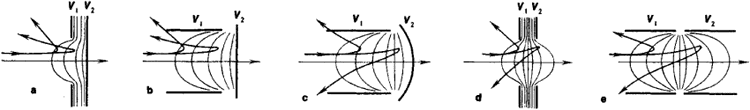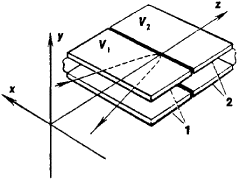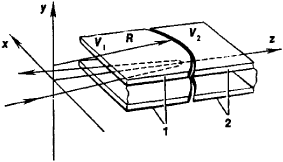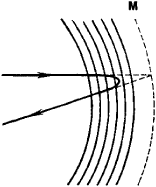# Electron Mirror

## electron mirror

[i′lek‚trän mir·ər]
(electronics)
McGraw-Hill Dictionary of Scientific & Technical Terms, 6E, Copyright © 2003 by The McGraw-Hill Companies, Inc.
The following article is from The Great Soviet Encyclopedia (1979). It might be outdated or ideologically biased.

## Electron Mirror

an electric or magnetic system that reflects electron beams and is designed either to produce electron-optical images by means of such beams or to change the direction of motion of electrons.

Electron mirrors essentially are systems that are symmetric with respect to some axis. Axially symmetric electrostatic mirrors (Figure 1) are used to create accurate electron-optical images of objects. If the last electrode of such an electron mirror is solid and if the electrons undergo a change in direction of motion in the immediate vicinity of the mirror’s surface, then a magnified image of the microscopic relief of the surface can be obtained. This property of electron mirrors is used in the electron mirror microscope.Figure 1. Axially symmetric two-electrode electron mirrors: (V1) and (V2) potentials of the electrodes. The thin lines are intersections of equipotential surfaces and the plane of the figure. The lines with arrows represent trajectories of electrons of different energies. Mirrors (a) and (b) are always diverging mirrors; mirrors (c), (d), and (e) may be either diverging or converging mirrors.Figure 2. Electrostatic cylindrical electron mirror: (1) and (2) electrodes with potentials V1 and V2, respectively. The term “cylindrical” may be applied to electron-optical systems because, when used as electron lenses, they can act on an electron beam just as a cylindrical optical lens acts on a pencil of light.

Cylindrical electron mirrors with a two-dimensional electric or magnetic field (Figure 2) that is independent of the x-coordinate are used to change the direction of electron beams. For electrons moving in the midplane of the mirror, the angle of incidence equals the angle of reflection, as is the case when a light beam is reflected from an optical mirror.Figure 3. Electrostatic transaxial electron mirror: (1) and (2) electrodes with potentials V1 and V2, respectively; (R) radius of curvature of the gap between the electrodes. The plane xz is superposed on the midplane of the mirror.

Transaxial electron mirrors (Figures 3 and 4) exhibit small aberrations (image errors) in the direction parallel to the midplane of the mirror.Figure 4. Reflection of a beam of electrons in the midplane of a transaxial electrostatic electron mirror. The solid lines are intersections of equipotential surfaces and the midplane of the mirror. The broken curved line represents the effective reflecting surface of the electron mirror, which corresponds to the reflecting surface of its optical analogue, mirror (M).

### REFERENCES

Glaser, W. Osnovy elektronnoi optiki. Moscow, 1957. (Translated from German.)
Kel’man, V. M., and S. Ia. Iavor. Elektronnaia optika, 3rd ed. Leningrad, 1968.

V. M. KEL’MAN and I. V. RODNIKOVA